# Fast Fourier Transform for polynomial multiplication

## Introduction

In this article, you will learn the idea of polynomial multiplication by fast Fourier transform.

Let me tell you that you need not know about Fourier transformation to understand this concept. Moreover, I will introduce the mathematical terms which are required in the algorithm for easy understanding.

Let's see the problem statement to understand our goal.

## Problem Statement

There are two polynomials given A(x) and B(x), Find a polynomial C(x) such that A(x)*B(x) = C(x).

Example -

Say, A(x) = x^2 + 2x + 3 and B(x) = 2x^2 + 5

Then C(x) = A(x)*B(x) can be calculated as -

This is a traditional approach that we follow. The time complexity for this method is O(n^2)

Is there a way to reduce the time complexity?

Yes. Polynomial multiplication using fast Fourier transform can be performed in O(nlogn) time.

## Representation of Polynomials

### Coefficient Representation

A coefficient representation of a polynomial of degree n-1 is a vector of coefficients

𝑎 = (𝑎0, 𝑎1, … , 𝑎𝑛−1) .The representation in the above example is called coefficient representation

### Point-Value Representation

There is another way to represent polynomials, and it is called point value representation. According to the fundamental theorem of algebra, any polynomial of degree n-1 can be uniquely defined by its evaluation at n distinct values of x or by n point value pairs.

Example

• The coefficient representation of A(x) = x^2 + 2x + 3 is (1,2,3).
• Degree of A(x) is 2.
• For 3 distinct values of x, we will get 3 values of A(x).
• So, if x0,x1 and x2 are the points considered, then let y0 = A(x0), y1=A(x1) and y2=A(x2).
• So, A(x) can be specified by the following 3 pairs - (x0,y0), (x1,y1) and (x2,y2).

Quick question: How does point-value representation help to multiply the polynomials faster?

If you have two polynomials A(x) and B(x) in their point-value representation, them to find C(x) = A(x)B(x), we can compute C(x) in point-value form by multiplying the corresponding values of A(x) and B(x) at distinct points.

So, you have -

• A(x) = {(x0, A(x0)),(x1, A(x1)), . . . ,(xn−1, A(xn−1))}
• B(x) =  {(x0, B(x0)),(x1, B(x1)), . . . ,(xn−1, B(xn−1))}
• Then, the point value form of C(x) will be - {(x0, A(x0)B(x0)),(x1, A(x1)B(x1)), . . . ,(xn−1, A(xn−1)B(xn−1))}, where the n distinct points are {x0,x1,x2,....xn-1}.

The time complexity seems to be linear as you have to multiply n corresponding values. But there is a catch!!

If A(x) and B(x) are of degree n-1 each, then their product will be of degree  n-1+n-12n-2. So, to represent C(x), we will need not n points but 2n-1 points. How to achieve this? Simple, we use extended point value representation of A(x) and B(x) by using 2n point value pairs instead of n

### Conversion from Point-value to coefficient form and vice-versa

The process of conversion from point-value form to coefficient form is called Interpolation.

Let’s summarize the steps for polynomial multiplication -

1. Given A(x) and B(x), find their point-value representation.
2. Compute C(x) = A(x)B(x) in point-value form.
3. Find coefficient representation of C(x) from its point-value representation.

We know that Step-2 takes linear time. So, now we aim to optimize the conversion between the two forms to multiply the polynomials efficiently.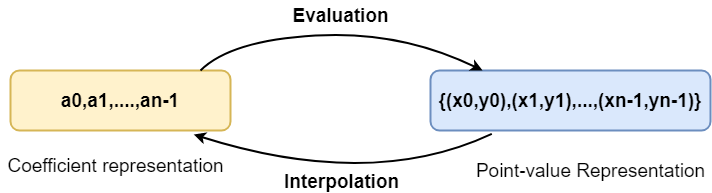### Time complexity

• In general, it takes Θ(n) operations for evaluating a polynomial at a single point x. As we have to evaluate at 2n points, so the total number of operations becomes 2n*(Θ(n)), i.e., Θ(n^2 ).

• Using fast Fourier transform, we can evaluate a polynomial on a set of 2n points by using only Θ(nlogn) operations as well as interpolation can also be done using Θ(nlogn) operations.

• The idea is to evaluate the polynomials not on any ordinary points but the complex roots of unity

## Fast Fourier Transform

It computes the Discrete Fourier transform of a polynomial in O(nlogn) time by using the properties of complex roots of unity.

### DFT(Discrete Fourier transform)

• Evaluation of a polynomial at n complex nth roots of unity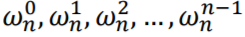is called discrete Fourier transform of the polynomial.
Here,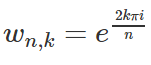• It is assumed that n is always a power of 2, and the polynomial A(x) is given in the coefficient form.
• The vector 𝑦 = (𝑦0, 𝑦1, … , 𝑦𝑛−1) where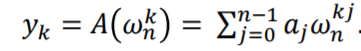for k=0,1,...n-1, is said to be the DFT of the coefficient vector 𝑎 = 𝑎0, 𝑎1, … , 𝑎𝑛−1 , denoted as 𝑦 = DFT𝑛(𝑎).

### Divide and Conquer strategy of FFT

The coefficient vector is divided into two vectors. Then we compute the DFT of these two vectors recursively and finally combine the result of these two to obtain the DFT of the initial polynomial.

Let’s see how FFT computes DFT in O(nlogn) time -

1. For a polynomial A(x), define a new polynomial of degree n/2, using even-index and odd-index coefficients of A, respectively.

2. We get -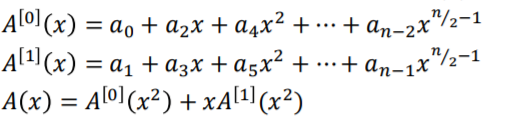3. Now, instead of evaluating the polynomial A at n distinct roots, we need to evaluate 𝐴 (𝑥) and A(x) at the points -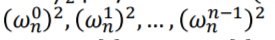4. Now, the above list does not contain n distinct values but n/2 complex n/2th roots of unity. (Observe that the problem size has been reduced by half).

5. This is nothing but recursion as we got two subproblems having the same form but are half the size of the main problem.

6. If we can compute DFT(A) from DFT(A0) and DFT(A1) in linear time, i.e. O(n) time, then the recurrence relation becomes-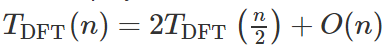7. By master theorem, we can prove that the time complexity is O(nlogn).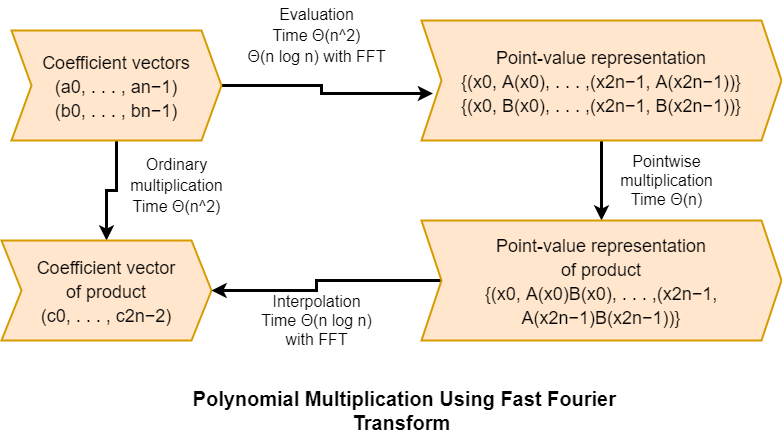Now, its time to see the recursive algorithm for FFT

1. For the polynomials, A(x) and B(x), first add n higher-order zero coefficients as we need 2n points for the product.

2. Evaluate A(x) and B(x) at 2n points using FFT.

3. Compute the point-value form of the product by multiplying A(x) and B(x).

4. Perform interpolation to get the coefficient form of the product/inverse DFT.

Let’s see the recursive code implementation for FFT in the next section.

## C++ Implementation

``````/*C++ code to compute DFT using FFT for fast multiplication of polynomials*/
#include <bits/stdc++.h>
using namespace std;

/*it stores the values of roots of unity*/
typedef complex<double> nth_rootsOfUnity;

// function to compute DFT using FFT
vector<nth_rootsOfUnity> fft(vector<nth_rootsOfUnity> &coefficientVec)
{
int n = coefficientVec.size();

// only one element is present
if (n == 1)
return vector<nth_rootsOfUnity>(1, coefficientVec);

// to store values of n complex nth roots of unity
vector<nth_rootsOfUnity> w(n);
for (int i = 0; i < n; i++)
{
double alpha = -2 * M_PI * i / n;
w[i] = nth_rootsOfUnity(cos(alpha), sin(alpha));
}

vector<nth_rootsOfUnity> A0(n / 2), A1(n / 2);
for (int i = 0; i < n / 2; i++)
{

// even indexed coefficients
A0[i] = coefficientVec[i * 2];

// odd indexed coefficients
A1[i] = coefficientVec[i * 2 + 1];
}

// recursion to compute DFT(A0)
vector<nth_rootsOfUnity> y0 = fft(A0);

// recursion to compute DFT(A1)
vector<nth_rootsOfUnity> y1 = fft(A1);

// to store the DFT vector y = { y0, y1, y2, ..., yn-1}
vector<nth_rootsOfUnity> y(n);

for (int k = 0; k < n / 2; k++)
{
y[k] = y0[k] + w[k] * y1[k];
y[k + n / 2] = y0[k] - w[k] * y1[k];
}
return y;
}

int main()
{
vector<nth_rootsOfUnity> coefficientVec{-1, 5, 10, 4};
vector<nth_rootsOfUnity> b = fft(coefficientVec);

cout << "The DFT of the coefficient vector is - \n";
for (int i = 0; i < 4; i++)
cout << b[i] << endl;

return 0;
}``````

Output

``````The DFT of the coefficient vector is -
(18,0)
(-11,-1)
(0,0)
(-11,1)``````

### Time Complexity

O(nlogn) and we have already discussed the computation of time complexity through recursive relation in previous sections.

### Space Complexity

Due to the recursive stack, the space complexity turns out to be exponential, which is O ((2^n)n). This is because the function is called twice in every recursion, and in total, an array of length 2^n needs to be stored times.

1. What is FFT?
The Fast Fourier Transform (FFT) is simply a fast (computationally efficient) way to calculate the Discrete Fourier Transform (DFT).

2. Is there any application of Fast Fourier transform for polynomial multiplication?
It can be used to multiply two long numbers in O(nlogn) time, where n is the number of digits.

## Key Takeaways

In this article, we learnt the idea of polynomial multiplication by fast Fourier transform right from basics to its implementation. There are many applications of fast Fourier transform in the field of computer science which you can explore.